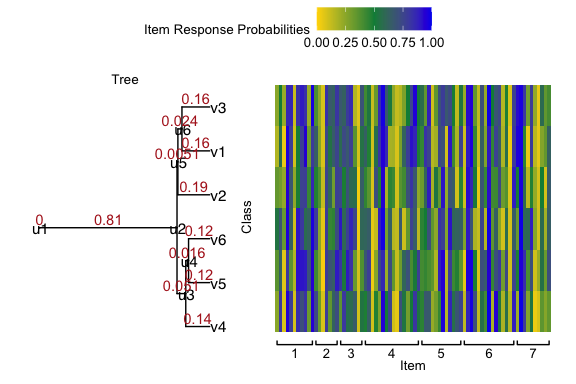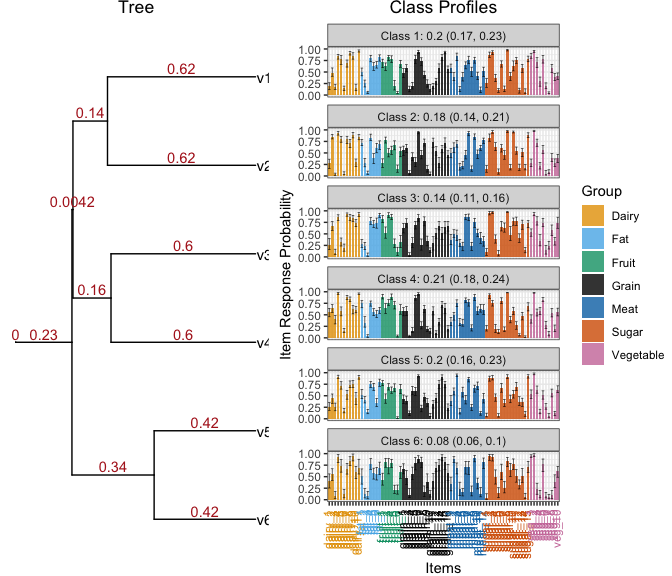# Vignettes for ddtlcm: An R package for fitting tree-regularized Bayesian latent class models

#### September 14, 2023

This is a simple vignette; please see here for the complete information.

# 1 Introduction

This document demonstrates the use of the ddtlcm package to fit Bayesian tree-regularized latent class models (LCMs) described in the manuscript “Tree-Regularized Bayesian Latent Class Analysis for Improving Weakly Separated Dietary Pattern Subtyping in Small-Sized Subpopulations”.

## 1.1 Summary of the paper

Dietary patterns synthesize multiple related diet components, which can be used by nutrition researchers to examine diet-disease relationships. Latent class models (LCMs) are widely used to analyze multivariate categorical food exposure data collected by dietary intake assessment tools such as dietary recalls. In LCMs, class profiles represent dietary patterns describing the probability of exposure to a set of food items. However, off-the-shell LCMs face unique limitations when deriving dietary patterns. First, dietary patterns are primarily distinguished only by a subset of food items when subjects share similar cultures and geographical location. In other words, dietary patterns are weakly separated from one another. Weak separation in class profiles may lead to unstable class profile estimates and less accurate class assignments, which are exacerbated in small-sized subpopulations. Second, the degree of separation of dietary patterns varies by pre-specified major food groups (e.g., sugar, fat, vegetables), which is ignored by commonly used Bayesian LCMs. Recognizing the distinct degrees of pattern separation by major food groups may not only improve dietary pattern estimation accuracy, but also embrace nutrition grouping information into dietary pattern interpretation. To resolve these challenges, we introduce . Our proposed model addresses weak separation under small sample sizes by (1) sharing statistical strength between classes guided by an unknown tree, and (2) integrating varying degrees of shrinkage across major food groups.

We develop a hybrid Metropolis-Hastings-within-Gibbs algorithm to sample from the posterior distribution of model parameters. Specifically, a Metropolis-Hastings (MH) step is designed to sample tree structures, and a Gibbs sampler with P'olya-Gamma augmentation to sample the LCM parameters, including probabilities of item exposure, latent class assignments, and class probabilities. The main algorithm is implemented via the ddtlcm_fit() function in R.

For posterior summaries, the tree structure is obtained via the (MAP) estimate from posterior samples. We compute posterior means as well as 95% credible intervals for the LCM parameters. Posterior summaries can be conducted using the summary() function.

## 1.2 Overview of the report

We look at a semi-synthetic data example of the Hispanic Community Health Study/Study of Latinos (HCHS/SOL). The full HCHS/SOL used in the manuscript is publicly available only by request, and thus we provide a simulated dataset micmicking our analysis cohort. We describe how to generate the data, fit the proposed model, and visualize the results. For the sake of time, this example runs the MCMC chain with fewer iterations than the manuscript. The total compilation time of this documents in 46 seconds on a 2018 Apple MacBook Pro.

We also describe how the simulation results of the manuscript can be reproduced using the codes. The results in this document will be produced using summary data rather than raw data, due to the extensive amount of run time of the numerical experiments in the manuscript. All models can be replicated exactly using the functions in the package on a high-performing computing cluster.

# 2 Installation and dependencies

The ddtlcm package can by installed by running the following command.

install.packages("devtools",repos="https://cloud.r-project.org")
devtools::install_github("limengbinggz/ddtlcm")

Throughout the document, we assume the working directory is where this R Markdown file is located.

# 3 A Semi-synthetic data example

We provide a detailed workflow of applying our model to a semi-synthetic dataset micmicking our analysis cohort in the Hispanic Community Health Study/Study of Latinos (HCHS/SOL).

## 3.1 Simulate data

We start with demonstrating how a semi-synthetic dataset is simulated using the parameters estimated from applying DDT-LCM to the real data. As described in the manuscript, our analysis cohort consists of $$N = 496$$ subjects and $$J = 78$$ food items. These food items are categorized into $$G = 7$$ pre-defined major food groups, including dairy, fat, fruit, grain, meat, sugar, and vegetables. The (MAP) tree estimate with $$K = 6$$ latent classes can be accessed via loading the data file “data_hchs” contained in the package. This data file contains the following objects:

• tree_phylo: a “phylo” object. The MAP tree estimated from the real HCHS/SOL data.

• item_membership_list: a list of $$G$$ elements, where the $$g$$-th element contains the column indices of the observed data matrix corresponding to items in major group $$g$$.

• item_name_list: a named list of $$G$$ elements, where the $$g$$-th element contains a vector of item names for items in item_membership_list[[g]]. The name of the $$g$$-th element is the name of the major item group. In the HCHS/SOL data, these names are dairy, fat, fruit, grain, meat, sugar, and vegetables.

• class_probability: a length-$$K$$ vector for the posterior mean class probabilities estimated from the real HCHS/SOL data.

• Sigma_by_group: a length-$$G$$ vector for the posterior mean group-specific diffusion variances.

library(ddtlcm)
# load the MAP tree structure obtained from the real HCHS/SOL data
data(data_hchs)
# unlist the elements into variables in the global environment
list2env(setNames(data_hchs, names(data_hchs)), envir = globalenv())
#> <environment: R_GlobalEnv>

# look at items in group 1
g <- 1
# indices of the items in group 1
item_membership_list[g]
#> []
#>    1  2  3  4  5  6  7  8  9 10 11
# names of the items in group 1. The name of the list element is the major food group
item_name_list[g]
#> $Dairy #>  "dairy_1" "dairy_2" "dairy_3" "dairy_4" "dairy_5" "dairy_6" #>  "dairy_7" "dairy_8" "dairy_9" "dairy_10" "dairy_11" For the purpose of illustration, we will simulate one semi-synthetic dataset, although the in manuscript we demonstrate simulation results using 100 replicates. # number of individuals N <- 496 # set random seed to generate node parameters given the tree seed_parameter = 1 # set random seed to generate multivariate binary observations from LCM seed_response = 1 # simulate data given the parameters sim_data <- simulate_lcm_given_tree(tree_phylo, N, class_probability, item_membership_list, Sigma_by_group, root_node_location = 0, seed_parameter = 1, seed_response = 1) The semi-synthetic data and its parameters are included in a named list sim_data, containing a “phylo4d” object of tree structure with node parameters sim_data$tree_with_parameter, a simulated $$496 \times 78$$ multivariate binary response matrix sim_data$response_matrix, and numeric vectors/matrices of LCM parameters. Let us look at how the tree and the generated data look like. response_matrix <- sim_data$response_matrix
dim(response_matrix)
#>  496  78
response_prob <- sim_data$response_prob tree_with_parameter <- sim_data$tree_with_parameter
plot_tree_with_heatmap(tree_with_parameter, response_prob, item_membership_list)On the left is the MAP tree estimated from the real HCHS/SOL data, with leaf nodes labeled “v1”, …, “v6” corresponding to latent classes 1 to 6. On the right is a heatmap of the simulated class profiles, where the 78 columns correspond to items with curly brackets underneath denoting the pre-specified major item groups.

## 3.2 Apply DDT-LCM

We assume that the number of latent classes $$K = 6$$ is known. Throughout the paper, we use divergence function $$a(t) = c / (1-t)$$ to parameterize the DDT branching process, where $$c > 0$$ is a hyperparameter. To use the function ddtlcm_fit, we need to specify the number of classes (K), a matrix of multivariate binary observations (data), a list of item group memberships (item_membership_list), and the number of posterior samples to collect (total_iters). In Section 5.1 of the manuscript, we set total_iters = 15000. For the purpose of quickly illustrating the output, here we run the function with a smaller number total_iters = 100.

set.seed(999)
# number of latent classes, or number of leaves on the tree
K <- 6
system.time({
result <- ddtlcm_fit(K = K, data = response_matrix,
item_membership_list = item_membership_list, total_iters = 100)
})
#>
#> ## Start posterior sampling ##
#> ## Finish posterior sampling
#>    user  system elapsed
#>  18.736   0.298  19.388

print(result)
#>
#> ---------------------------------------------
#> DDT-LCM with K = 6 latent classes run on 496 observations and 78 items in 7 major groups.
#> 100 iterations of posterior samples drawn.
#> ---------------------------------------------

We next summarize the posterior chain by discarding the first 50 iterations as burn-ins (burnin = 30). To deal with identifiability of finite mixture models, we perform post-hoc label switching using the Equivalence Classes Representatives (ECR) method by specifying relabel = T. To save space in the document, we do not print the summary result here (be_quiet = T).

burnin <- 50
summarized_result <- summary(result, burnin, relabel = T, be_quiet = T)

We can visualize the summarized result, including the MAP tree and the class profiles. Due to limited space, the item labels are scrambled together. Please refer to Figure 4 in the manuscript for a clearer version.

plot(x = summarized_result, item_name_list = item_name_list, plot_option = "all")Using the summarized result, we can compute the RMSE of the posterior mean item response probabilities to assess the quality of the estimates.

rmse <- sqrt(mean((summarized_result$response_probs_summary[, "Mean"] - sim_data$response_prob)**2))
cat("\nRMSE of the item response probabilities:", rmse)
#>
#> RMSE of the item response probabilities: 0.2029731

Next, we can predict individual class memberships using two methods. First, the predicted class memberships can be obtained from the modal assignments calculated from posterior summaries.

predicted_class_assignments1 <- predict(summarized_result, response_matrix)
cat("\nFrequencies of predicted class memberships from posterior summaries:\n", tabulate(predicted_class_assignments1$class_assignments, K)) #> #> Frequencies of predicted class memberships from posterior summaries: #> 93 89 67 99 106 42 The second method is to predict class memberships from the posterior predictive distribution, which is more computationally intensive. predicted_class_assignments2 <- predict(result, response_matrix, burnin) cat("\nFrequencies of predicted class memberships from posterior predictive distribution:\n", tabulate(predicted_class_assignments2$class_assignments, K))
#>
#> Frequencies of predicted class memberships from posterior predictive distribution:
#>  94 88 66 104 104 40